# Bihar Board Class 9th Maths Solutions Chapter 6 Lines and Angles Ex 6.2

Bihar Board Class 9th Maths Solutions Chapter 6 Lines and Angles Ex 6.2 Textbook Questions and Answers.

## BSEB Bihar Board Class 9th Maths Solutions Chapter 6 Lines and Angles Ex 6.2Question 1.
In figure, find the values of x and y and then show that AB || CD.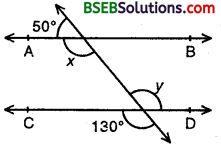Solution:
Since AB || CD and transversal PQ intersects them at R and S respectively.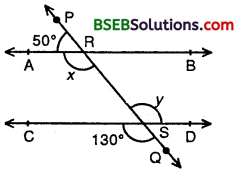∴ ∠ARS = ∠RSD [Alternate angles]
⇒ x = y
But ∠RSD = ∠CSQ
[ Vertically opp. angles]
⇒ y = 130° [∵∠CSQ = 130°]
Hence, x = y = 130°.

Question 2.
In figure, if AB || CD, CD || EF and y : z = 3 : 7, find x.Solution:
Since CD || EF and transversal PQ intersects them at S and T respectively.
∠CST = ∠STF [Alternate Angles]
⇒ 180° – y = z
[∵ ∠y + ∠CST = 180° being linear pair]
⇒ y + z = 180°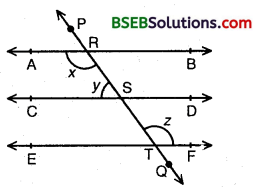Given y : z = 3 : 7.
So, the sum of ratios = 3 + 7 = 10
∴ y = $$\frac { 3 }{ 10 }$$ x 180°
= 3 x 18° = 54°
and, z = $$\frac { 7 }{ 10 }$$ x 180°
= 7 x 18° = 126°
Since AB || CD and transversal PQ intersects them at R and S respectively.
∴ ∠ARS + ∠RSC = 180° [Consecutive interior angles are supplementary]
⇒ x + y = 180°.
⇒ x = 180° – y
= 180° – 54° = 126° [∵ y = 54°]
Hence x = 126°.Question 3.
In figure, if AB || CD, EF ⊥ CD and ∠GED = 126°, find ∠AGE, ∠GEF and ∠FGE.Solution:
Since AB || CD and transversal GE cuts them at G and E respectively.
∠AGE = ∠GED [Alternate angles]
∠AGE = 126° [∵ ∠GED = 1260 (given)]
∠GEF = ∠GED – ∠FED = 126° – 90° = 36°
∠FGE = ∠GEC [Alternate angles]
∠FGE = 90° – ∠GEF
= 90° – 36° = 54°
Hence, ∠AGE = 126°, ∠GEF = 36° and ∠FGE = 54°.

Question 4.
In figure, if PQ || ST, ∠PQR = 110° and ∠RST =130°, find ∠QRS.Solution:
Produce PQ to intersect SR in a point M.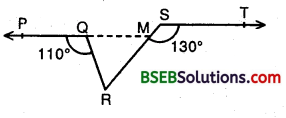Now, PM || ST and transversal SM intersects them at M and R respectively.
∴ ∠SMQ = ∠TSM [Alternate angles]
⇒ ∠SMQ = 130°
⇒ ∠QMR = 180° – 130° = 50° [∵ ∠SMQ + ∠QMR = 180° linear pairs]
Since, ray RQ stands at Q on PM.
∴ ∠PQR + ∠RQM = 180°
⇒ 110° + ∠RQM = 180°
⇒ ∠RQM = 70°
∴ ∠QRS = 180° – (70° + 50°) = 60°
[∵ Sum of the angles of a triangle is 180°]

Question 5.
In figure, if AB || CD, ∠APQ = 50° and ∠PRD = 127°, find x and y.Solution:
∵ AB || CD and transversal PQ intersects them at P and Q respectively.
∴ ∠PQR = ∠APQ [Alternate angles]
⇒ x = 50° [∵ ∠APQ = 50° (given)]
∵ AB || CD and transversal PR intersects them at P and R respectively.
∴ ∠APR = ∠PRD [Alternate angles]
⇒ ∠APQ + ∠QPR = 127° [∵ ∠PRD = 127°]
⇒ 50° + y = 127° [∵∠APQ = 50°]
⇒ y = 127° – 50° = 77°
Hence, x = 50° and y = 11°.Question 6.
In figure, PQ and RS are two mirrors placed parallel to each other. An incident ray AB strikes the mirror PQ at B, the reflected ray moves along the path BC and strikes the mirror RS at C and again reflects back along CD. Prove that AB || CD.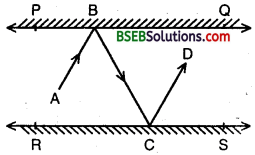Solution:
Two-plane mirrors PQ and RS are placed parallel to each other i.e., PQ || RS. An incident ray AB after reflections takes the path BC and CD.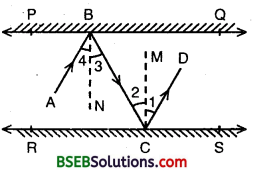BN and CM are the normals to the plane mirrors PQ and RS respective.
Since BN ⊥ PQ, CM ⊥ RS and PQ || RS
∴ BN ⊥ RS ⇒ BN || CM
Thus BN and CM are two parallel lines and a transversal BC cuts them at B and C respectively.
∴ ∠2 = ∠3 [Alternate interior angles]
But, ∠1 = ∠2 and ∠3 = ∠4 [By laws of reflection]
∠1 + ∠2 = ∠2 + ∠2 and ∠3 + ∠4= ∠3 + ∠3
⇒ ∠1 + ∠2 = 2(∠2) and ∠3 + ∠4 = 2(∠3)
⇒ ∠1 + ∠2 = ∠3 + ∠4 [∵ ∠2 = ∠3 ⇒ 2(∠2) = 2(∠3)]
⇒ ∠ABC = ∠BCD
Thus, lines AB and CD are intersected by transversal BC such that
∠ABC = ∠BCD.
i.e., alternate interior angles are equal.
Therefore, AB || CD.